## Optical Fiber Classification:

The Optical Fiber Classification of light transmission through a glass fiber depend on many factors, for example:

3. #### The diameter and length of the fiber

The composition of the fiber determines the refractive index. By a process called doping, other materials are introduced into the material that alter its index number. This process produces a single fiber with a core index n1 and a surface index (cladding) n2 (typically n1 = 1.48 and n2 = 1.46).

Another characteristic of the Optical Fiber Classification, which depends on its size, is its mode of operation. The term “mode” as used here refers to mathematical and physical descriptions of the propagation of energy through a medium. The number of modes supported by a single fiber can be as low as 1 or as high as 100,000; that is, a fiber can provide a path for one light ray or for hundreds of thousands of light rays. From this characteristic come the terms single mode and multi-mode. These Optical Fiber Classification are illustrated in Figure 18-17. For long-haul communications only single-mode fiber cables are used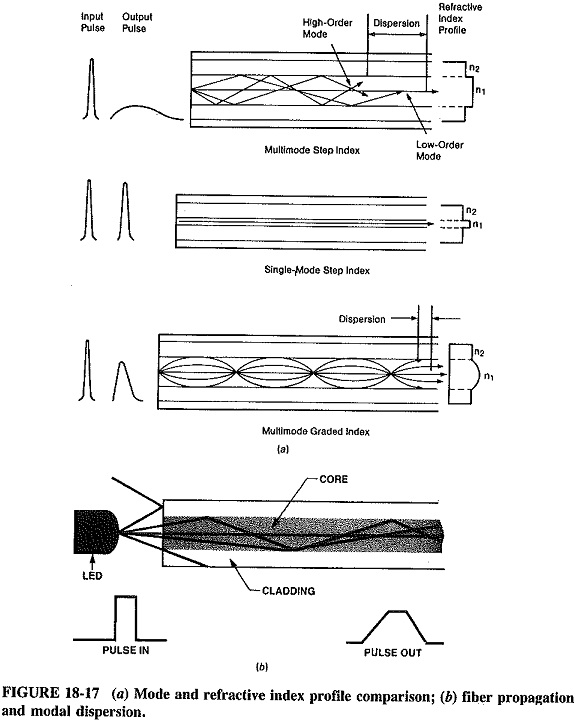Another term which should be mentioned here is the refractive index profile. It describes the relationship between the multiple indices which exist in the core and the cladding of the particular fiber. This relationship can be expressed in simple terms by the statement “Light changes speed when it passes from one medium to another.” There are two major indices in this relationship:

1. #### Step index

The step index describes an abrupt index change (see Table 18-1) from the core to the cladding, for example, a core with a uniform index (1.48) and a cladding with a uniform index (1.46). With graded-index fiber, the highest index is at the center (1.48). This number decreases gradually until it reaches the index number of the cladding (1.46), that is, near the surface.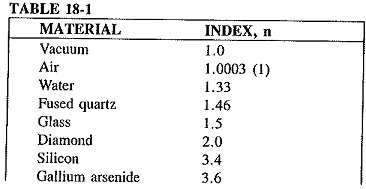From these terms come three Optical Fiber Classification:

3. #### Single-mode step-index fiber

The multimode step-index fiber has a core diameter of from 100 to 970 jam. With this large core diameter, there are many paths through which light can travel (multimode). Therefore, the light ray traveling the straight path through the center reaches the end before the other rays, which follow a zigzag path. The difference in the length of time it takes the various light rays to exit the fiber is called modal dispersion. This is a form of a signal distortion which limits the bandwidth of the fiber.

The multimode graded-index fiber is an improvement on the multimode step-index fiber. Because light rays travel faster through the lower index of refraction, the light at the fiber core travels more slowly than the light nearer the surface. Therefore, both light rays arrive at the exit point at almost the same time, thus reducing modal dispersion (an example of these losses can be seen in Figure 18-17). A typical graded-index fiber has core diameters ranging from 50 to 85 μm and a cladding diameter of 125 μm.As previously mentioned, single-mode step-index fibers are the most widely used in today’s wide-band communication arena. With this fiber alight ray can travel on only one path; therefore modal dispersion is zero. The core diameters of this fiber range from 5 μm to 10 μm (standard cladding diameter is 125 μm). The extra cladding thickness tends to set an overall fiber size standard and makes the fiber less fragile (refer to Figure 18-18 for composition). Some specifications for a single-mode fiber are:

5. #### The mode field diameter (MFD; spot size) is larger than the core diameter.

Numerical aperture (NA) relates to the light-gathering capabilities of a fiber. Only light that strikes the fiber at an angle greater than the critical angle (θc) will be propagated. The NA relates to the indices of both the core and the cladding; that is,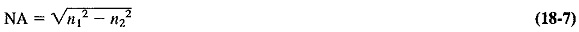From Equation (18-7) we can develop another relationship which also describes the maximum light propagation angle; it is commonly called the cone of acceptance (see Figure 18-19).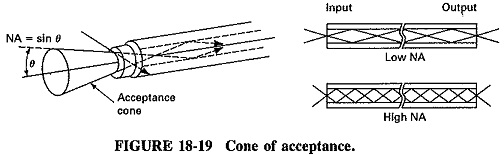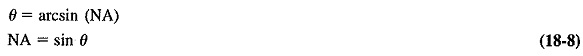In general, Optical Fiber Classification with high bandwidths have low NA and thus fewer modes and less modal dispersion. NAs range from 0.50 for plastic to 0.21 for graded-index fibers.

Scroll to Top